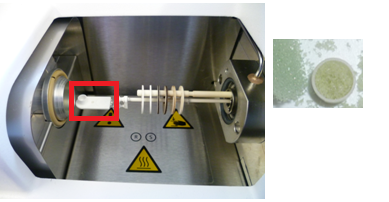# Any Progress on inverse pyrolysis modelling

## with ensemble learning methods?

 Patrick Lauer University of Wuppertal lauer@uni-wuppertal.de

## What is pyrolysis?

Burning of solids can be separated in two phases:

• Thermochemical decomposition of solid material and phase change from solid to gas phase (Pyrolysis)
• Chemical reaction in the gas phase (Combustion)

To predict fire spread, we need to model burning of solids, hence pyrolysis.

### How do we model pyrolysis?

Boundary condition

(1)

Heat conduction

(2)

Reaction rate:

(3)

### Parameter overview

Parameter
Activation energie
Pre-exponential factor
Reaction order
Density
Conduction coefficient
Heat capacity
Emissivity
Heat of reaction

### How do we get these parameters?

Find parameters with small scale experiments and mathematical fitting, scale up to parts and devices

Usual experiments:

• Thermogravimetrical analysis
• Cone calorimeter
• Micro combustion calorimeter

### Exkurs: Thermogravimetrical analysis (TGA)

 A small specimen (mg scale) gets heated in a furnace with a constant or transient heating rate. Mass loss of the specimen is captured. It allows to estimate reaction kinetics of this sample.Figure 2. TGA

### Approaches

• Basic graphical fitting [4, 5, 8]
• Inverse modeling 
• Optimization algorithms [1, 7]
• Machine learning

## Forests of randomized trees

Common Methods:

• Random Forests 
• Extremly Randomized Trees 

Concept

• Ensemble learning methods

• Efficient for big data sets
• Fast to train
• Easy to parallelize

## Method

• Train a model to predict reaction kinetic parameters with given reaction rate
• Case study: mockup TGA experiment with constant heating rate
• All data used is randomly generated with the pyrolysis model

### Process I

1. Generating sample data set with the regarding model
• Samples for 1, 2 and 3 reactions, with 3 heating rates applied each
• Up to 1M samples generated, with and ,

### Exkurs: Sampling I

Problem:

• Arrhenius equation is an exponential function
• might be
• might be
• Hence we can't sample from a uniform distribution

Challenge:

• Find a distribution to sample from

### Exkurs: Sampling II

Solution:

• Introducing (reference temperature), (reference range) and (reference rate), characterizing a triangle of temperature at maximum mass loss rate and width of the peak

### Exkurs: Sampling III

• and are sampled from a uniform distribution and then mapped to and
• Mapping between , and , , is done with these equations:
(4)
(5)
(6)

### Process II

1. Splitting data set in two independent sets (75 % training data set and 25 % validation data set)
2. Train model with training data set
• Input:
• Output: ,
• Model adopts to transform input to output
3. Validate trained model by feeding of validation data set and check for expected outcome

### Process III

1. Recalculate with , , calculate RMSE between and

### Process IV

1. Evaluate
2. Repeat with different algorithms and different hyperparameter settings

## Results

for inverse replacement models with

• 1 reaction, 3 heating rates,
• 2 reactions, 3 heating rates and
• 3 reactions, 3 heating rates

built with Extremly Randomized Trees algorithm